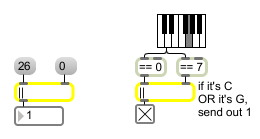# ||

If either of two numbers is non-zero, output a 1

## Description

|| takes any given number, compares it to another specified number, and outputs a 1 if either of the two numbers are non-zero numbers, or a 0 if both numbers are 0.

## Arguments

Name Type Opt Description
initial-value int opt Sets an initial value to be stored by ||. A number in the right inlet changes the value set by the argument.

## Messages

 bang In left inlet: Performs the operation with the numbers currently stored. If there is no argument, || initially holds 0. int input [int] If the number in either inlet is not 0, then the output is 1. If the number in both of the inlets is 0, then the output is 0. A number in the left inlet triggers the output. (inlet1) comparison-value [int] In right inlet: The number is compared to the number in the left inlet to determine output but only a number in the left inlet will trigger output. float input [float] Converted to int. set set-input [int] The word set followed by a number will set the input value without causing output.

## Output

int: If either the number in the left inlet or the number in the right inlet (or specified by the argument) is not 0, then the output is 1. Otherwise, the output is 0.

## ExamplesOne of the numbers is not 0... Used to combine comparisons